# NCERT Solutions for Class 11 Chemistry Structure of Atom Part 1## myCBSEguide App

CBSE, NCERT, JEE Main, NEET-UG, NDA, Exam Papers, Question Bank, NCERT Solutions, Exemplars, Revision Notes, Free Videos, MCQ Tests & more.

NCERT solutions for Class 11 Chemistry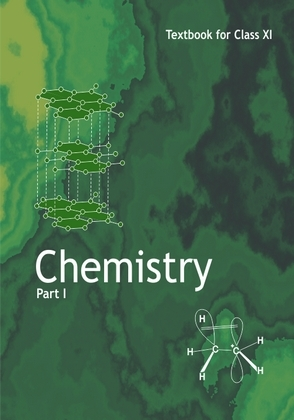## NCERT Class 11 Chemistry Chapter wise Solutions

• Chapter 1 – Some Basic Concepts of Chemistry
• Chapter 2 – Structure of Atom
• Chapter 3 – Classification of Elements and Periodicity in Properties
• Chapter 4 – Chemical Bonding and Molecular Structure
• Chapter 5 – States of Matter
• Chapter 6 – Thermodynamics
• Chapter 7 – Equilibrium
• Chapter 8 – Redox Reactions
• Chapter 9 – Hydrogen
• Chapter 10 – The s-Block Elements
• Chapter 11 – The p-Block Elements
• Chapter 12 – Organic Chemistry – Some Basic Principles and Techniques
• Chapter 13 – Hydrocarbons
• Chapter 14 – Environmental Chemistry

## NCERT Solutions for Class 11 Chemistry Structure of Atom Part 1

Chapter 2 – Class 11 Chemistry Structure of Atom Part 1
1. (i) Calculate the number of electrons which will together weight one gram.

(ii) Calculate the mass and charge of one mole of electrons.

2. (i) Calculate the total number of electrons present in one mole of methane.

(ii) Find (a) the total number and (b) the total mass of neutrons in 7 mg of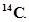(Assume that mass of a neutron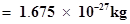).

(iii) Find (a) the total number and (b) the total mass of protons in 34 mg of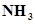at STP.

Will the answer change if the temperature and pressure are changed?

3. How many neutrons and protons are there in the following nuclei?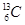,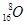,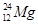,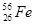,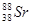4. Write the complete symbol for the atom with the given atomic number (Z) andAtomic mass (A)

(i) Z = 17, A = 35

(ii) Z = 92, A = 233

(iii) Z = 4, A = 9

Ans. (i)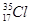(ii)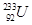(iii)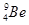5. Yellow light emitted from a sodium lamp has a wavelength (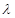) of 580 nm. Calculate the frequency (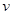) and wave number (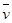) of the yellow light.

6. Find energy of each of the photons which

(i) correspond to light of frequency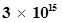Hz.

(ii) have wavelength of 0.50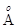.

7. Calculate the wavelength, frequency and wave number of a light wave whose period is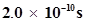.

8. What is the number of photons of light with a wavelength of 4000 pm that provide 1 J of energy?

9. A photon of wavelength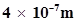strikes on metal surface, the work function of the metal being 2.13 eV. Calculate (i) the energy of the photon (eV), (ii) the kinetic energy of the emission, and (iii) the velocity of the photoelectron (1 eV=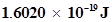).

10. Electromagnetic radiation of wavelength 242 nm is just sufficient to ionise the sodium atom. Calculate the ionisation energy of sodium in kJ mol-1.

11. A 25 watt bulb emits monochromatic yellow light of wavelength of 0.57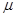m. Calculate the rate of emission of quanta per second.

12. Electrons are emitted with zero velocity from a metal surface when it is exposed to radiation of wavelength 6800. Calculate threshold frequency (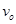) and work function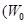) of the metal.

13. What is the wavelength of light emitted when the electron in a hydrogen atom undergoes transition from an energy level with n = 4 to an energy level with n =2?

14. How much energy is required to ionise a H atom if the electron occupies n = 5 orbit? Compare your answer with the ionization enthalpy of H atom (energy required to remove the electron from n =1 orbit).

15. What is the maximum number of emission lines when the excited electron of an H atom in n = 6 drops to the ground state?

16. (i) The energy associated with the first orbit in the hydrogen atom is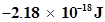atom-1. What is the energy associated with the fifth orbit?

(ii) Calculate the radius of Bohr’s fifth orbit for hydrogen atom.

## NCERT Solutions for Class 11 Chemistry Chapter 2 Structure of Atom Part 1

NCERT Solutions Class 11 Chemistry Structure of Atom Part 1 PDF (Download) Free from myCBSEguide app and myCBSEguide website. Ncert solution class 11 Chemistry includes text book solutions from both part 1 and part 2. NCERT Solutions for CBSE Class 11 Chemistry have total 14 chapters. 11 Chemistry NCERT Solutions in PDF for free Download on our website. Ncert Chemistry class 11 solutions PDF and Chemistry ncert class 11 PDF solutions with latest modifications and as per the latest CBSE syllabus are only available in myCBSEguide

## NCERT Solutions for Science Class 3rd to 12th

To download NCERT Solutions for class 11 Chemistry, Physics, Biology, History, Political Science, Economics, Geography, Computer Science, Home Science, Accountancy, Business Studies and Home Science; do check myCBSEguide app or website. myCBSEguide provides sample papers with solution, test papers for chapter-wise practice, NCERT solutions, NCERT Exemplar solutions, quick revision notes for ready reference, CBSE guess papers and CBSE important question papers. Sample Paper all are made available through the best app for CBSE students and myCBSEguide.com website.